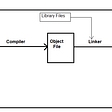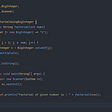# Overview

In this blog, we will learn to create a simple BMI calculator in Java programming language where the BMI value is calculated based on the inputs given by the user in metric units.

# What is BMI?

BMI stands for Body Mass Index. It is used to measure the body mass based on the height and weight of the individual. The range of BMI classifies individuals as underweight, normal or overweight. The main BMI categories are shown below:

# Formula for Calculating BMI in Metric Units

The formula for calculating BMI value is:

BMI = Weight in Kg/(Height in Meters * Height in Meters)

For example, let us consider height = 1.5m and weight = 90kg

BMI = 90 / (1.5 * 1.5) = 40 (Obese).

# How It Works?

1. Input the weight in kg and height in cm from the user.
2. A static method CalculateBMI() is called by passing height and weight as its arguments.
3. The BMI value is calculated by dividing weight in kg with square of the height in meters.
4. The height in centimeters can be converted to height in meters by dividing the height value by 100 (1 m = 100 cm).
5. The obtained BMI value is displayed on the console.
6. Also, if-else statements are used to categorize the BMI values. So, according to the obtained BMI value, the if-else statements will be executed.

# Source Code

Here is the complete source code for Java program that calculates the BMI value based on the inputs given by the user in metric units. Also, note that the height input is to be given in centimeters.

# Output

Here is a sample output of the above program.

Love podcasts or audiobooks? Learn on the go with our new app.## Kubernetes Deployment and Testing Strategies## Google T5 Translation as a Service in Just 7 lines of Codes## EPISODE #60: Why Lasting Automation Success and Competitive Advantage Require Process Excellence## How to add a Github third-party authentication to your Rails MVC## Migration Success!## Reset phase at Encora Apprenticeship— Week 1## ABSTRACTION IN JAVA## Why Java is platform independent## How to find factorial of any number in java..